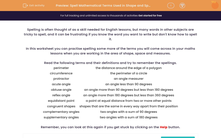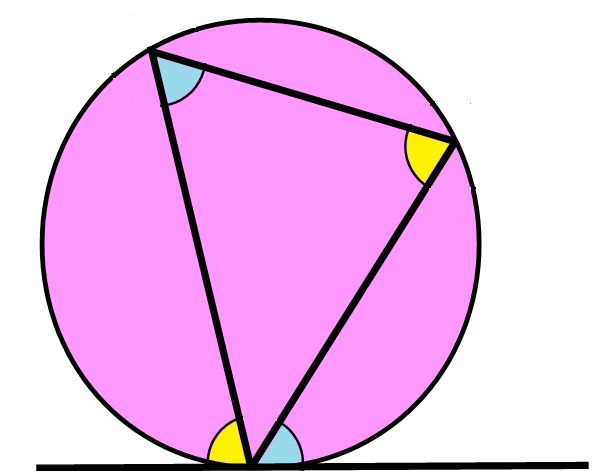# Spell Mathematical Terms Used in Shape and Measure

In this worksheet, students will practise spelling terms related to shape and measure.Key stage:  KS 3

Curriculum topic:   Grammar and Vocabulary

Curriculum subtopic:   Vocabulary and Grammar Awareness

Difficulty level:#### Worksheet Overview

Spelling is often thought of as a skill needed for English lessons, but many words in other subjects are tricky to spell, and it can be frustrating if you know the word you want to write but don't know how to spell it.

In this activity, we will practise spelling some more of the terms you will come across in your maths lessons when you are working in the area of shape, space and measures.Read the following terms and their definitions and try to remember the spellings.

 Perimeter The distance around the edge of a polygon Circumference The perimeter of a circle Protractor An angle measurer Acute angle An angle less than 90 degrees Obtuse angle An angle more than 90 degrees but less than 180 degrees Reflex angle An angle more than 180 degrees but less than 360 degrees Equidistant point A point at equal distance from two or more other points Congruent shapes Shapes that are the same in every way apart from their position Complementary angles Two angles with a sum of 90 degrees Supplementary angles Two angles with a sum of 180 degrees

Remember, you can look at this again if you get stuck by clicking on the red help button.